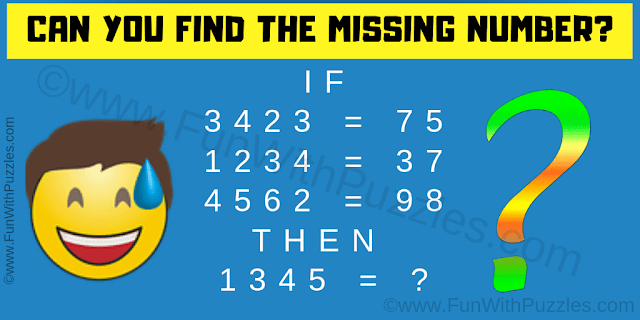It is very interesting and easy logic math question which will tickle your brain. Think like a kid to solve this math logic puzzle question. In this puzzle question, there are some logical equations. Find the logic used in these equations and then solve the last equation. So can you find the missing number?Can you solve the Logic Math Question?
Answer of this "Logic Math Question", can be viewed by clicking on the answer button.